Covid-19 Update: We've taken precautionary measures to enable all staff to work away from the office. These changes have already rolled out with no interruptions, and will allow us to continue offering the same great service at your busiest time in the year.

# Analysis of Approaches to Bargaining Models

3916 words (16 pages) Essay in Economics

23/01/18 Economics Reference this

Disclaimer: This work has been submitted by a student. This is not an example of the work produced by our Essay Writing Service. You can view samples of our professional work here.

Any opinions, findings, conclusions or recommendations expressed in this material are those of the authors and do not necessarily reflect the views of UK Essays.

Abstract

This paper discusses the various types of approaches to bargaining models, namely indifference curves and iso-profit curves, monopoly union behaviour and efficient contracts. Then we go on to study the concept of efficiency wages in a unionised as well as a non-unionised environment and with the help of existing economic theories we develop a model and incorporate the alternative wage rate. On completion of this paper, we will be able to understand the influence of wage level, alternative wage rate and other factors on the employment level, which would be highly important to both the firms as well as the labourer’s side while framing policies.

Introduction

Before starting with the paper, we need to know what efficiency wages are. It is the wage that is set by the firms or the employers which is higher than the market clearing wage. There are certain implications behind this action. Doing this, it would encourage workers’ loyalty towards the employer; the firms would be able to attract higher number of talents and thereby improving the applicants’ pool, raise the morale of the workers and as a result the overall efficiency of the firms increases. In various efficiency wage models, labour productivity has a positive relationship with the wage rate. Also worth mentioning, is that the efficiency wage model is an extension from the Shapiro-Stiglitz model of efficiency wage. In this paper, we combine both the microeconomics concept of labour union and the model of Shapiro-Stiglitz to derive the various propositions.

Moving ahead, we discuss the basic two models of wage rate determination for the unionised and non-unionised sector of the economy. The first would be the monopoly model, as prescribed by Oswald in 1985, assumes that the labour union sets the wage and the employer chooses the profit maximizing employment level. The second case also stated by Oswald in 1985, notes that both the employer’s side as well as the worker’s side can benefit from the monopoly outcome by jointly bargaining over the wages and employment level.

Literature Review

• Oswald, A. (1985): “The Economic Theory of Trade Unions: An Introductory Survey” Scandinavian Journal of Economics, volume 87.

Oswald assumed that the union sets the wage and the employer chooses the profit maximising employment level. He also stated that the efficient bargaining model notes that both sides can improve on the monopoly outcome by jointly bargaining over wages and employment.

• Brown, J. and Ashenfelter, O (1986, June): “Testing the Efficiency of Employment Contracts” Journal of Political Economy, volume 94.

They used the significance of a measure of alternative wages in an employment regression as evidence for the efficient bargaining model.

• Stiglitz, J. (1987, March): “The Causes and Consequences of the dependence of quality on price” Journal of Economic Literature, volume 25.

In relation to the efficiency wages hypothesis, Stiglitz stated that, “one motivation for this literature is to explain involuntary unemployment: If the efficiency wage is framework is valid, then firms may not lower wages even in the face of excess supplies of labour.

• Krueger, A. and Summers, L. (1988, March): “Efficiency wages and the inter-industry wage structure” Econometrica, volume 56

Another additional motivation of this literature is the that the empirical observation that inter-firm or inter-industry wage differentials remain even after most possible economic determinants of these differentials have been controlled.

• Katz, L. and Summers, L. (1989): “Industry rents: Evidence and Implications” Brookings Papers on Economic Activity, Microeconomics.

The wage differentials tend to lower quits and increases the length of queues of job seekers attempting to gain entry. They explained the relationship between the existences of rents associated with efficiency wages.

Research Question

• What is the effect of general wage level and alternative wage rate on the employment level, when efficiency wages are paid both in a non-union as well as union setting?

Methodology

The theory of income distribution is the study of the determination of the shares of the factors of production in the total output produced in the economy over a given period of time. For simplicity, we assume two factors of production, labour and capital, their shares are defined as follows:

Share of Labour = (w*L)/ X and share of capital = (r*K)/X

Where w= wage rate, r= rental of capital, L=quantity of labour employed, K=quantity of capital employed and X=value of output produced in economy.

With this backdrop, we proceed on to the model where we consider firms and labours perspective, in both unionised and non-unionised labour setting. Initially, labour force is unionised. As a union, three of the most commonly pursued goals are: maximization of employment, maximization of total wage bill and maximization of total gains to the union as a whole. The general conclusions derived from this microeconomic thought are firstly, if the firm buyers have no monopsonistic power, labour unions can possibly attain an increase in the wage rate at the cost of a lower level of unemployment. Secondly, if the firm buyers have monopsonistic power, the unions actions can eliminate one part of the monopsonistic exploitation and thirdly, if the firm buyers have monopsonistic power, trade unions can increase the total wage bill in most of the cases, by either increasing employment or the wage rate or both.

Considering, the concept of efficiency wage hypothesis and incorporating the alternative wage rate as used by Shapiro and Stiglitz we combine this macroeconomic phenomenon with the microeconomic concept of labour union. Looking at the employment level, alternative wage rate, normal wage rate we can run a regression analysis on the employment level with various other variables and determine the significance of these and come up with propositions under different cases.

Bargaining Models

In the context of labour unions, there are different types of bargaining that can take place between a firm and a labour union. These methods are also applicable in many other aspects other than labour unions.

• Indifference Curves and Iso-profit Curves

Here, we look at the union’s preferences as the preference for a single worker. We can formulate the utility of the worker as a function of consumption, C and leisure, L, i.e. U (C, L). Representing, the utility function in terms of wage rate, w and labour supplied, h, we can write it as follows:

U (h, w) = U (w*h, 1-h)where C = w*h and considering time constraint L= 1 – h. An indifference curve in (h, w) space is defined by setting u as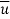(constant) and we define w implicitly as a function of h, w (h). Therefore, we can write it as follows:

U (h, w (h)) = U (w (h)*h, 1-h) =Differentiating, the above equality with respect to h and hence obtaining the slope of the indifference curve.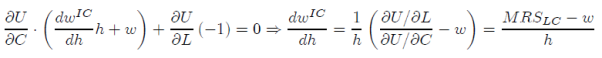This implies that along the labour supply curve, where MRS = w the indifference curve will have zero slope. To the left of the labour supply curve, workers work more and so MRS < w and the indifference curve is downward sloping. Symmetrically, to the right MRS > w and the indifference curve is upward sloping. We can reinterpret the first order condition for finding labour supply as the worker finding the highest indifference curve in (w, h) subject to the constraint that w equals the offered wage, leading to the tangency shown below.

Looking at the firm’s side, its preferences are derived using the iso-cost curve. The firm’s profit function can be written as follows:

Π (E, w) = f (E) – w*E

We set the price to unity and along an iso-profit curve, we set the profit equal to some constant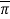, which implies an implicit relationship between w and E. Therefore, we can write it as f (E) – w (E)*E =.

Differentiating, the above equation implicitly, we find the slope of the iso-profit curve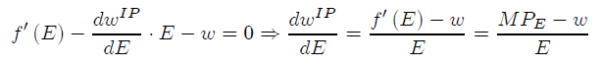along the demand curve MPE = w, implying that iso-profit curves are flat when they cross the labour demand curve. Left of the demand curve, means MPE > w hence iso-profit curve is upward sloping, and right of the labour demand curve, means MPE < w hence iso-profit curve is downward sloping.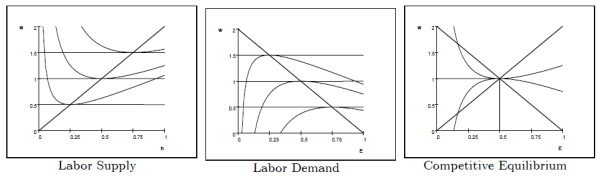• Monopoly union Bargaining

In this model, the labour union sets the wage rate, w and the firm chooses the employment level, E. Since, the firm’s objective is to maximize profits, it will set the employment level at the point where VMPE = w. Assuming the union acts like a single individual so that h = E, its problem is then

Max U (w*E, 1- E)subject to MPE = w

Maximizing with respect to E, and using the first order conditions we get, f’ (E) = w.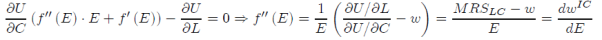The above expression implies that the indifference curve will have a negative slope while the iso-profit curve has a zero slope and to interpret the cross of the two curves it would mean inefficiency. Workers would be willing to work more at a slightly lower wage and firms would make profits hiring them. However even if unions do function this way, that does not mean they are necessarily bad – workers are made better off, but these gains are smaller than the losses to firms and consumers. If the value of the redistribution to workers is considered more important than the loss to the other parties then the union may still be a “good” thing. However it would be better for everyone if the union and firm could find a more efficient way of bargaining.

• Efficient Contracts

This is another model of unions which assumes that the labour union and firm will bargain in such a way that it leads to an efficient outcome. Now, any Pareto efficient outcome will be reached between two parties by guaranteeing some level of profits to the firm,and maximizing the union’s utility.

Max U (w*E, 1- E)subject to f (E) – w*E =On solving, we get w = (f (E) –) / E. The first order condition can be written as follows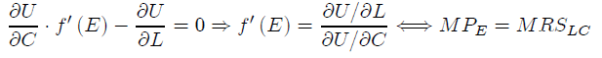Solving algebraically we get that the iso-profit curve and the indifference curves are tangent. It cannot be solved as to which combination of (E, w) will be chosen as there are several points- the locus of all these points represent the contract curve. Some information on profit and utility functions is necessary to determine whether the contract curve of the efficient contracts is downward or upward sloping, or vertical (the strongly efficient case).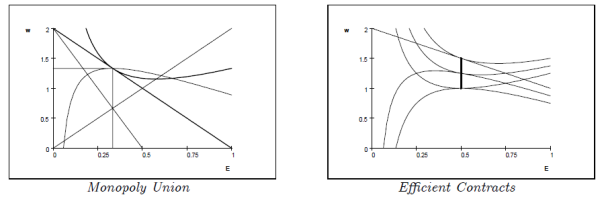The Model

General Assumptions:

• All the workers are identical.
• The worker’s choose their own level of work effort and this work effort is monitored by the firm with the help of technology.
• The monitoring process by the firm is not the most efficient or it is not perfect.
• The monitoring process can be expressed in terms of work effort as follows, q (e),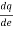>0, which implies that a worker will not be dismissed for an exogenously given level of work effort.
• All the workers have an identical utility function given as follows:

U (w, e) = w – e2(eqn. 1)

• The workers are provided with unemployment insurance or they can obtain another or alternative job with wage rate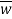.

Efficiency Wages in a non-union setting

Analysis:

Now, if the workers are able to choose their level of work effort, which is not monitored perfectly by the firm, then the firm may pay wages above the market wage rate to ensure a higher level of efficiency or effort by the worker. The question is how would alternative wages enter an employment regression in this case?

We have already assumed that the firm’s monitoring process can be expressed as a function of, q (e), suggesting that the workers are not dismissed for an exogenously given level of work effort. The workers can reduce their likelihood of getting dismissed, by the firm, by increasing their level of work effort. Implication behind this statement suggests that, q’ >0.

Let n be the elasticity of q with respect to level of effort. We can therefore show that the optimal effort for the worker is

e =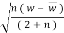(eqn. 2)

In order to model the firm, we make another assumption of a concave revenue function, f’’ < 0. This revenue function depends on efficiency of labour or the effective labour, eL, where L is the amount of labour hired. Therefore, the profit function of the firm can be written as

Π = f (e*L) – w*L(eqn. 3)

Using the optimization technique, the firm chooses the level of w and L, subject to the worker’s choice of e.

From the equations 2 and 3, we find out that the optimal wage rate, w is twice that of the alternative wage rate,.

Expressing f’ as a logarithmic form as a linear combination of various exogenous variables that affect the revenue and effective units of labour, the optimal amount of labour for the non-union firm is

ln L =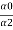+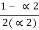ln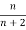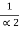ln w +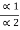X +ln (w –)

And ln f’ = α0 + α1X – α2 ln(e*L)(eqn. 4)

X is the vector of non-labour factors affecting the marginal revenue product of labour.

Interpretation of equation 4, is that the alternative wage rate,, conditional on w and X, will be negatively correlated with the actual or observed employment.

Proposition: On running a regression of employment on wage level and alternative wage rate, it should yield a negative coefficient for the alternative wage if efficiency wages are paid even in the absence of efficient bargaining.

Efficiency Wages in a Union setting

Here, we discuss the case for efficiency wages in a unionised scenario and find the resulting demand for labour under both (a) monopoly unions and (b) efficient bargaining methods.

1. Monopoly Unions

Considering that the union comprises total of N number of workers, who are employed at the wage rate, 2. Using, the previous method discussed we calculate the optimal worker effort, e*, where

e* =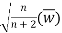Each worker faces the probability of getting dismissed with a probability of q (e*). We also assume that the workers getting dismissed by the firms are replaced immediately. Now, the union’s objective is to choose w, so as to maximize the expected utility, V, of unionised worker. Let L be the employment level at the new union wage, w. Then for each wage, w, we have,

V =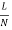[ q (w – e2) ] +if L < N

And V = q (w – e2) +if L ≥N (eqn. 5)

Now, in the case for monopoly unions, as the union raises the wage levels, it generally lowers the total employment level, hence we have L < N. Although, at some wages below 2, a rising w would lead to rising employment because of increased work effort. The union balances the negative effect of wages on employment and positive effects of wages on employed members’ utility. Multiplying, equation 5 by N, the union chooses w to maximize

V = Lq ((w – e2) (eqn. 6)

Subject to f’e = w

Using the optimization techniques, we solve for the monopoly union wage, w

w =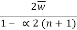(eqn. 7)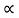2 is the measure of the slope or the steepness of the marginal revenue product curve. Higher the elasticity, n, with respect to effort, higher will be the union wage. In this model, the marginal revenue productivity condition for the monopoly model with efficiency wages is similar to the condition for non-union firms, although in this case, the unions will raise the wages and lower the total employment. This leads to the following proposition.

Proposition: Under monopoly model and efficiency wages, if we run a regression of employment on X, w andand a union shift term, the coefficient on the union shift should be zero. However, in a regression that includes only the exogenous variables X andand a union shift term, the coefficient should be negative.

1. Efficient Bargaining

Here, we focus on the case where labour and the management jointly set wage rate, w and employment level, L.

According to Mc. Donald and Solow, 1981, to derive the set of efficient contracts, they have suggested the necessary conditions for the contract curve.

Vw / VL = πw / πL

The subscripts represent the partial derivatives.

Using equations 3 and 6, and substituting in the above contract curve relation, we get,

(w – f’e) / (1 – f’ew) =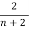(w –) > 0 (eqn. 8)

As long as the union raises the wages above the non-union wage 1- f’ew > 0 and so is w – f’e. Wages exceed the marginal revenue product of labour (as already suggested by McDonald and Solow, 1981). Algebraically, solving the slope of contract curve is not possible and hence is indeterminate which leads to the next proposition.

Proposition: Under efficient bargaining method and efficiency wages, if we run a regression of employment on X, w andand a union shift term, it will yield a positive coefficient for the union shift term as compared to a zero coefficient under monopoly model. However, in a regression that includes only the exogenous variables X and, the sign of the union shift coefficient is ambiguous, as compared to a negative coefficient in the monopoly model.

Conclusion

The results from the above classification of models suggests that traditional way of determining wage bill, i.e. labour times the wage rate, by the labour union and the employment level determination by the firm side are not the only factors that affect the decision making process of both the sides. Rather, the alternative wage rate, which is one of the factors taken up by Shapiro and Stiglitz in their “efficiency wage model”, is also instrumental in affecting the employment level. Another union shift term incorporated while running the regression, we find that it is also one of the determinants of employment determination. So, the ultimate conclusion that we can derive is that there are certain other factors as well in both wage and employment determination and these factors are statistically significant in different cases which again lead to various policy implications. Hence, modification of the theoretical microeconomic foundation and including certain other variables will show us a greater and deeper understanding of the employment determination and thereby various other policy prescriptions that both the sides can take into account while framing one.

References

• Stiglitz, J. (1976, July): “The Efficiency Wage Hypothesis, Surplus Labour and the Distribution of Income in L.D.C.s” Oxford Economics Papers, pp.185-207.
• Oswald, A. (1985): “The Economic Theory of Trade Unions: An Introductory Survey” Scandinavian Journal of Economics, volume 87.
• Brown, J. and Ashenfelter, O (1986, June): “Testing the Efficiency of Employment Contracts” Journal of Political Economy, volume 94.
• Katz, L. and Summers, L. (1989): “Industry rents: Evidence and Implications” Brookings Papers on Economic Activity, Microeconomics.
• Krueger, A. and Summers, L. (1988, March): “Efficiency wages and the inter-industry wage structure” Econometrica, volume 56
• Stiglitz, J. (1987, March): “The Causes and Consequences of the dependence of quality on price” Journal of Economic Literature, volume 25.
• Cowell, F.A. (2004, December): “Microeconomics: Principles and Analysis” STICERD and Department of Economics, London School of Economics.
• Autor, D.H. (2003, November): “Lecture Note: Efficiency Wages, Shapiro-Stiglitz Model” MIT and NBER.
• Koutsoyiannis, A. (1979): “Modern Microeconomics” Macmillan.

1

If you need assistance with writing your essay, our professional essay writing service is here to help!

## Related Services

View all

### DMCA / Removal Request

If you are the original writer of this essay and no longer wish to have the essay published on the UK Essays website then please:

Related Services